Gravity Wave Model

Tensor Up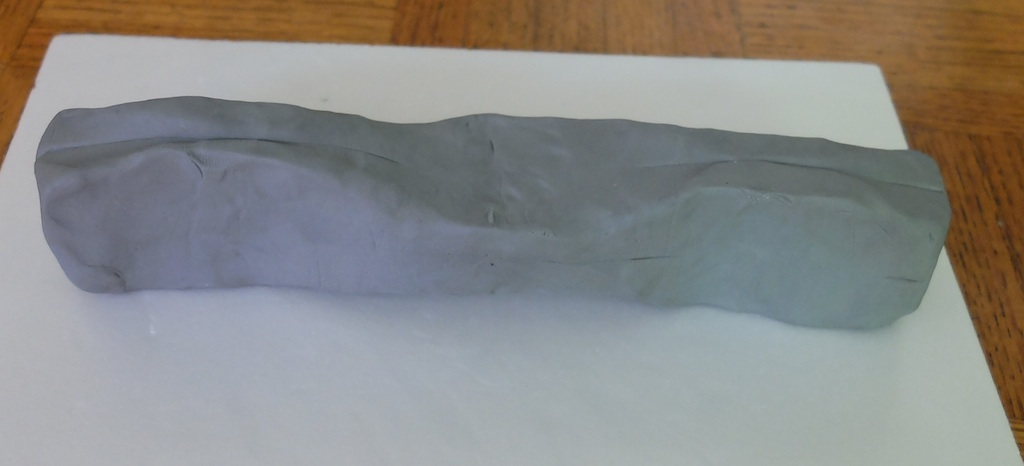A model of a gravity wave in clay

Introduction

Use clay to model a gravity wave.

Material

• Clay or Playdoh
• a pastic cylinder

To Do and Notice

Mash the clay into a rectangular beam with a square cross section.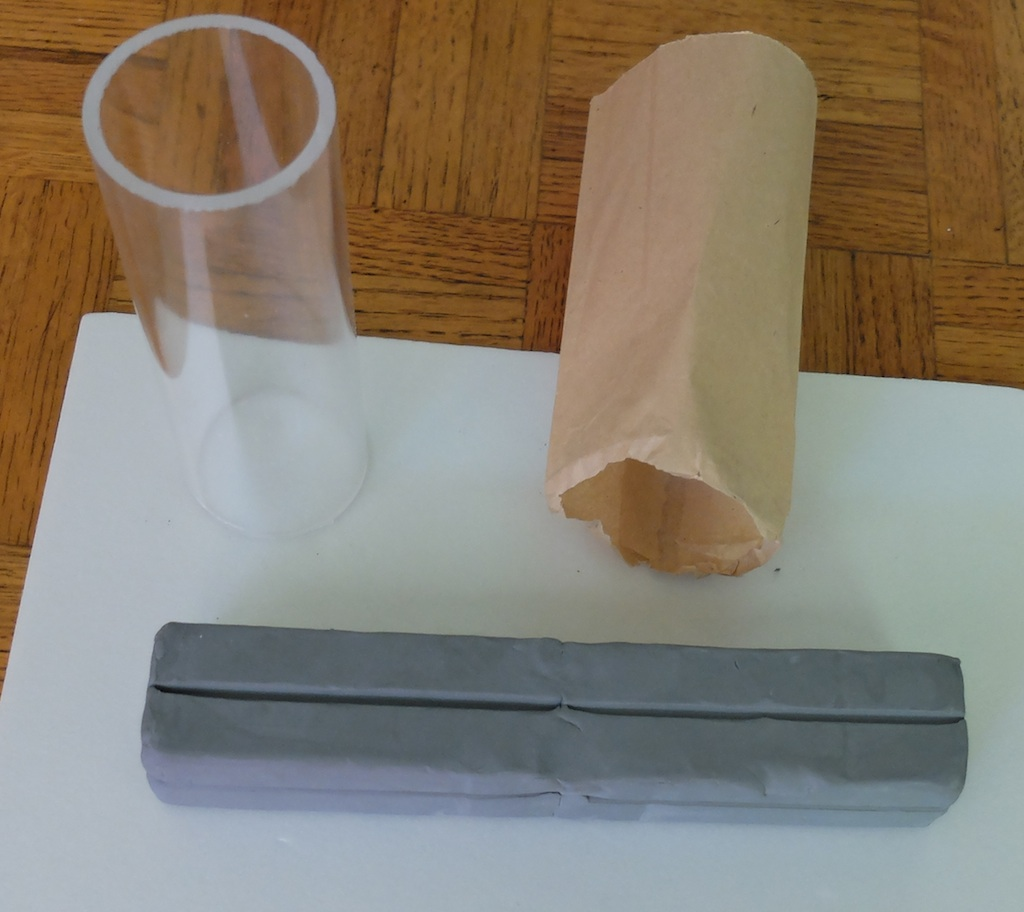Find a cilinder with a diameter that is 1/4 to 1/3 of the length of the clay beam.

If you care about the finish of your plastic cylinder, wrap it in paper.

Starting at one end of the clay use the cylinder to push into the clay to create a thinner yet wider region of the clay.

Then trotate the clay 90 degrees and push in both sides to make another thinner but wider region.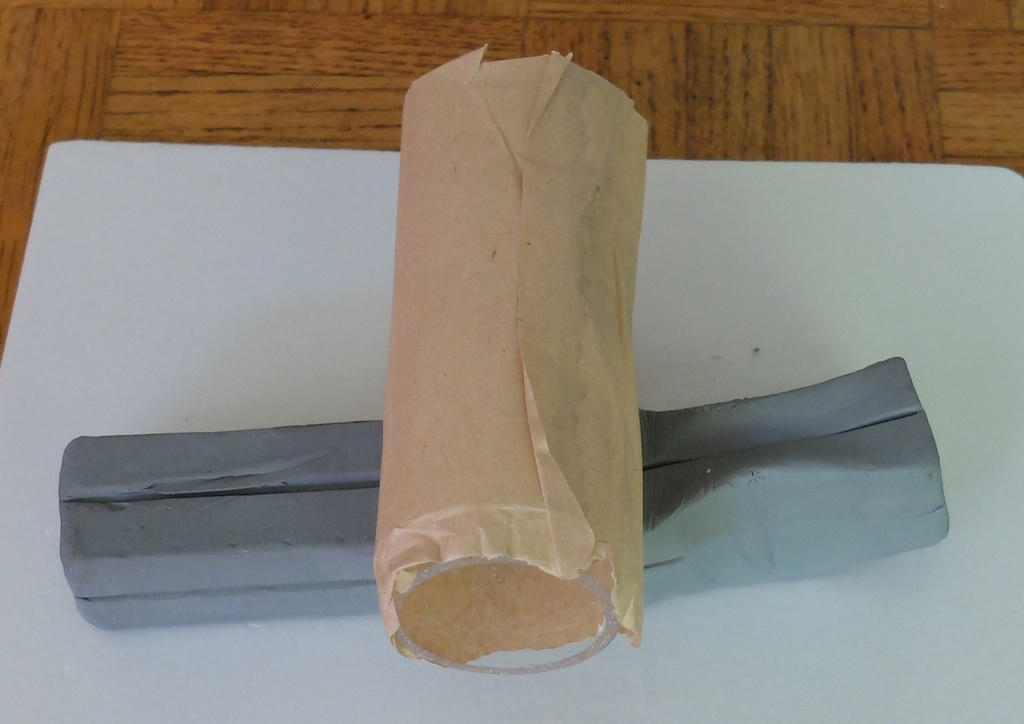Keep turning 90 degrees and squeezing in/widening the clay until you reach the end of the beam.

Smooth things out with your fingers, see the image at the top of this snack.

What's Going On?

A gravity wave is a tensor wave.

As it passes through the vacuum of space it stretches space in one direction and compresses it at right angles.

As a wave it oscillates between compression and expansion as it travels at the speed of light.

Math Root

A one dimensional wave might be represented by the density of gas along a line in a sound wave.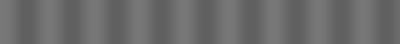Density, d, is just a number. The number might vary sinusoidally along the wave as it moves at the speed of sound v in the x direction where t is time.

d(x,t) = d0 sin( 2pi t/T - 2pi x/L) where T is the period and L is the wavelength of the sound wave. v = L/T

This could be modeled by a string with changing gray tones.

A vector wave might be the electric field in a light wave where the wave travels at c the speed of light in the x direction while the electric field E points in the y direction, j is a unit vector in the y direction.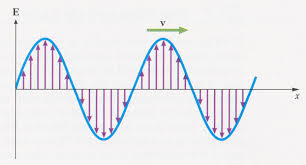E(x,t) = E0 sin (2pi t/T - 2pi x/L) j where the speed of light c is L/T

This could be modelled by arrows drawn on a card.

The gravity wave is a tensor wave.

 0 Gx(z,t) = G0 sin(2pi t/T-2pi z/L) 0 Gy(z,t) = G0 cos(2pi t/T-2pi z/L)

Etc.

LIGO The Laser Interferometric Gravitationalwave Observatories are searching for gravitational waves.

 Scientific Explorations by Paul Doherty © 2014 3 May 2014